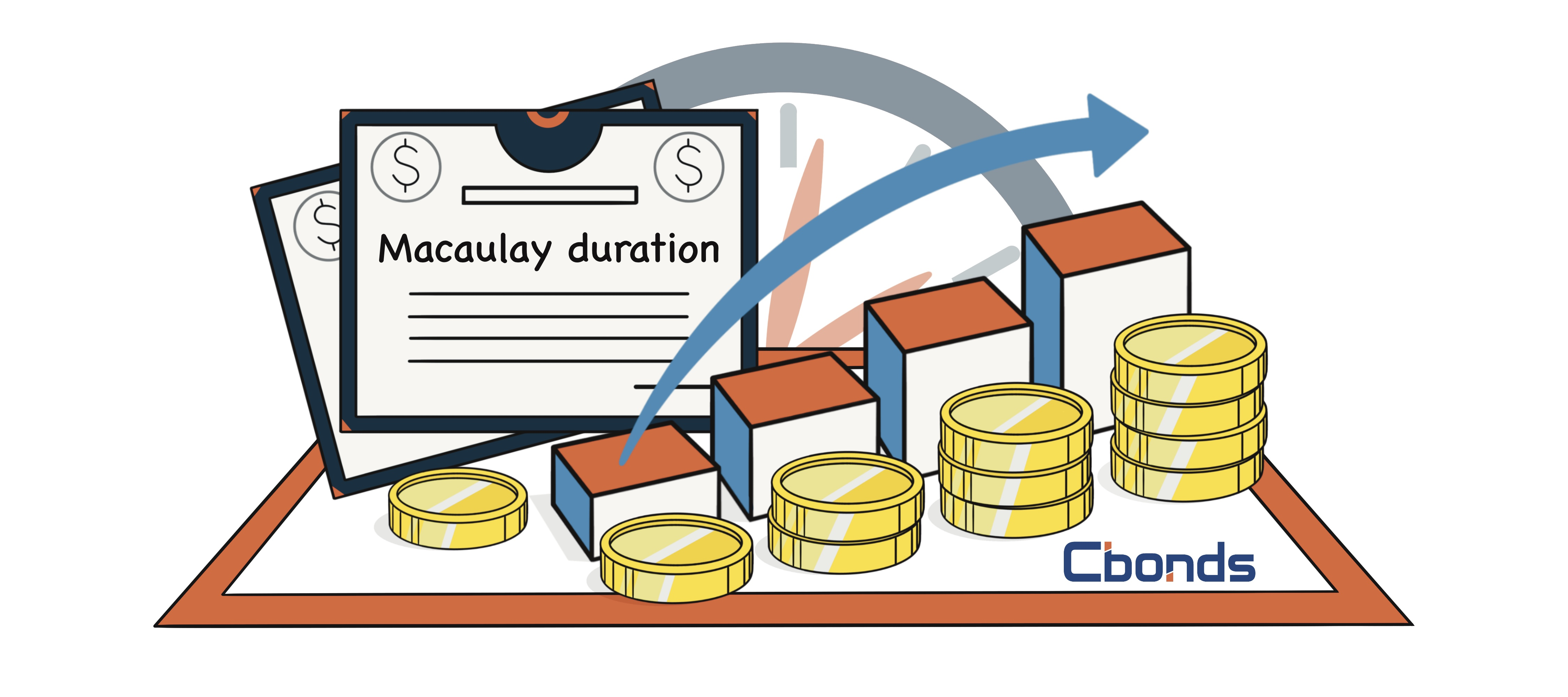Hint mode is switched on
Glossary

# Macaulay duration

Category — Analytical Metrics

## What is the Macaulay duration?

The Macaulay duration represents the average time until a bond’s cash flows are received and is calculated as the weighted sum of their maturities. Each cash flow’s weight is determined by dividing its present value by the bond’s price. Portfolio managers employing an immunization strategy often rely on Macaulay duration as a crucial metric.## What does Macaulay duration tell you?

The metric derives its name from its originator, Frederick Macaulay. It serves as the economic equilibrium point for a set of cash flows. Alternatively, the statistic represents the average duration an investor needs to hold a bond until the total present value of its cash flows equals the bond’s purchase price.

## How is Macaulay duration calculated?

To calculate the Macaulay duration, you multiply each time period by its corresponding periodic coupon payment and then divide the result by 1 plus the periodic yield raised to the time to maturity.

D = 1 + 1 / YTM

This calculation is performed for each period, and the values are then added together to obtain the Macaulay duration.

The duration calculator employs an annual compounding period to standardize duration values across various bonds. Duration is typically expressed in years in international markets (e.g., Bloomberg), while in the Russian and Ukrainian markets, it is mostly measured in days.

Beyond merely reflecting the average payment flow timeline of bonds, duration serves as a reliable indicator of price sensitivity to changes in interest rates.

## Example

The calculation of Macaulay duration is a straightforward process. Let’s consider a \$1,000 face-value bond with a 6% coupon, maturing in three years, and subject to a 6% per annum interest rate with semiannual compounding. The bond pays its coupon twice a year and the principal at the end. Here are the expected cash flows over the next three years:

Period 1: \$30

Period 2: \$30

Period 3: \$30

Period 4: \$30

Period 5: \$30

Period 6: \$1,030

To compute the discount factor for each period, use the formula 1 / (1 + r)^n, where r is the interest rate, and n is the period number. With a 6% interest rate compounded semiannually (3% per period), the discount factors are as follows:

Period 1 Discount Factor: 1 / (1 + 0.03)^1 = 0.9709

Period 2 Discount Factor: 1 / (1 + 0.03)^2 = 0.9426

Period 3 Discount Factor: 1 / (1 + 0.03)^3 = 0.9151

Period 4 Discount Factor: 1 / (1 + 0.03)^4 = 0.8885

Period 5 Discount Factor: 1 / (1 + 0.03)^5 = 0.8626

Period 6 Discount Factor: 1 / (1 + 0.03)^6 = 0.8375

Next, calculate the present value of each cash flow by multiplying the period’s cash flow by the period number and its corresponding discount factor:

Period 1: 1 × \$30 × 0.9709 = \$29.13

Period 2: 2 × \$30 × 0.9426 = \$56.56

Period 3: 3 × \$30 × 0.9151 = \$82.36

Period 4: 4 × \$30 × 0.8885 = \$106.62

Period 5: 5 × \$30 × 0.8626 = \$129.39

Period 6: 6 × \$1,030 × 0.8375 = \$5,175.65

Summing up the present values of all cash flows: \$29.13 + \$56.56 + \$82.36 + \$106.62 + \$129.39 + \$5,175.65 = \$5,579.71

Finally, calculate the Macaulay Duration by dividing the sum of the present values by the bond’s price: Macaulay Duration = \$5,579.71 ÷ \$1,000 = 5.58

The result, 5.58 half-years, is less than the time to maturity of six half-years, which equates to 2.79 years. Thus, the bond’s duration is indeed less than its time to maturity, as expected for a coupon-paying bond.

## Macaulay Duration vs. Modified Duration

Macaulay duration represents the weighted average time to maturity of a bond’s cash flows.

Modified duration, on the other hand, quantifies a bond’s price sensitivity to fluctuations in interest rates. It is derived from Macaulay duration but considers the bond’s yield to maturity (YTM) in its calculation.

## Setbacks of Using Duration

In asset-liability portfolio management, duration matching serves as a method for interest rate immunization. Variations in interest rates influence the present value of cash flows and, consequently, impact the value of a fixed-income portfolio. By aligning the durations of assets and liabilities in a company’s portfolio, a change in interest rates will cause the value of assets and liabilities to move by the exact same amount but in opposite directions.

As a result, the total value of the portfolio remains constant. However, it’s important to note that duration matching has its limitations. While it immunizes the portfolio against minor interest rate changes, it becomes less effective when dealing with significant fluctuations.

Get access
×

## — We have everything you need:full data on over 700 000 bonds, stocks & ETFs; powerful bond screener; over 350 pricing sources among stock exchanges & OTC market; ratings & financial reports; user-friendly interface; available anywhere via Website, Excel Add-in and Mobile app.

×

## WhyYou will have detailed descriptive & pricing data for 650K bonds, 76K stocks, 8K ETFsGet full access to the platform from any device & via Cbonds app